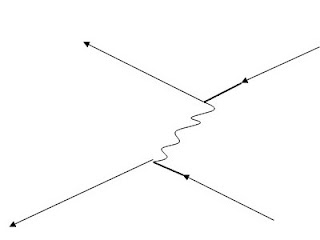## ΒΑΡΥΤΗΤΑ ΚΑΙ ΕΠΙΤΑΧΥΝΣΗΑπευθύνεται σε μαθήτριες και μαθητές του Λυκείου,Πραγματεύεται τις κοινές διαπιστώσεις παρατηρητή όταν βρεθεί μέσα σε κινούμενο ανελκυστήρα ή μέσα σε βαρυτικό πεδίο.

## Σάββατο 5 Μαρτίου 2011In feynman diagram, imagine that I have the interaction of two positrons! It's just a scattering of positrons, which has been studied about energy and momentum, which are the only measurements carried out without affecting the reaction. In this interaction we certainly particles entering and leaving the particles. Behind the yellow ball of ignorance,

I might have two nodes which describes a physical process most likely

from below, that if you disclose there are six. For every natural process, there is an infinite number of Feynman diagrams which describe. The probability that a given diagram describing the particular process falls within a particular factor for each particle of the body contains. The sum of all probabilities of diagrams that can describe the actual physical process is equal to the probability that the process is carried out. In an electromagnetic interaction of the virtual photon describes the transport of energy and momentum between loads. Each diagram describes a particular mathematical relationship, and each virtual photon decreases the probability of occurrence at 18769 fores.Next diagrams with many photons are unlikely to occur and thus they contribute little to the total sum.